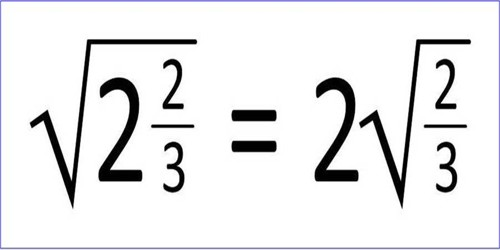Mathematic

# Comparison of SurdsDefinitions of surds: A root of a positive real quantity is called a surd if its value cannot be exactly determined. It is a number that can’t be simplified to remove a square root (or cube root etc). For example, each of the quantities √3, ∛7, ∜19, (16)^2/5 etc. is a surd.

More Examples:

• √2 (square root of 2) can’t be simplified further so it is a surd
• √4 (square root of 4) CAN be simplified to 2, so it is NOT a surd

Comparison of Surds

In the comparison of surds, we will discuss the comparison of equiradial surds and comparison of non-equiradical surds.

In case of equiradical surds (i.e., surds of the same order) n√a and n√b, we have n√a > n√b when x > y.

For example,

(i) √5 > √3, since 5 > 3

(ii) ∛21 < ∛28, since 21 < 28.

(iii) ∜10 > ∜6, since 10 > 6.

In case of comparison between two or more non-equiradical surds (i.e., surds of different orders) we express them to surds of the same order (i.e., equiradical surds). Thus, to compare between ∛7 and ∜5 we express them to surds of the same order as follows:

Clearly, the orders of the given surds are 3 and 4 respectively and LCM Of 3 and 4 is 12.

Therefore, ∛7 = 71/3 = 74/12 = 12√74 = 12√2401 and

∜5 = 51/4 = 53/12 = 12√53 = 12√125

Clearly, we see that 2401 > 125

Therefore, ∛7 > ∜5.

Example of comparison of surds:

Convert each of the following surds into equiradical surds of the lowest order and then arrange them in ascending order.

∛2, ∜3 and 12√4

Solution:

∛2, ∜3 and 12√4

We see that the orders of the given surds are 3, 4 and 12 respectively.

Now we need to find the lowest common multiple of 3, 4 and 12.

The lowest common multiple of 3, 4 and 12 = 12

Therefore, the given surds are expressed as equiradical surds of the lowest order (i.e. 12th order) as follows:

∛2 = 21/3 = 24/12 = 12√24 = 12√16

∜3 = 31/4 = 33/12 = 12√33 = 12√27

12√4 = 41/12 = 12√41 = 12√4

Therefore, equiradical surds of the lowest order ∛2, ∜3 and 12√4 are 12√16, 12√27and 12√4 respectively.

Clearly, 4 < 16 < 27; hence the required ascending order of the given surds is:

12√4, ∛2, ∜3.

Information Source: